# OliNo

Renewable Energy

## KLV Ledverlichting _ KLV_LISTRIP_RGB test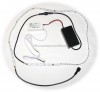presents a ledstring consisting of 30 RGB leds. With help of a controller the color of these leds is changed constantly. This is not something that could be stopped, so the measurement done has an averaged result of a lot of colors that changed during the measurement time. Some measurements were done several time to show the impact on this. These measurement results cannot be compared with the regular ones.

This article shows the measurement results. Many parameters are also found in the Eulumdat file.

See this overview for a comparison with other light bulbs.### Summary measurement data

parameter meas. result remark
Color temperature n.a. K No correlated olor temperature could be measured as very often one color is sofar from the Plackian locus that a CCT cannot be calculated.
Luminous intensity Iv 8.9 Cd Measured straight underneath the lamp.
Illuminance modulation index 83 % Measured straight underneath the lamp. Is a measure for the amount of flickering.

Note: this value depends on the color emitted. Some examples measured were 8, 22, 44, 83 %.

Beam angle 141 deg 141º for the C0-C180-plane (perpendicular to the length direction of the strip) and 134º for the C90-C270 plane, which is along the length direction of the lamp.
Power P 2.5 W The measured value depended on the color emitted and varied between 1.9 – 3.2 W.
Power Factor 0.44 For every 1 kWh net power consumed, there has been 2.1 kVAhr for reactive power.
THD 78 % Total Harmonic Distortion
Luminous flux 29 Lm This is an average value.
Luminous efficacy 12 Lm/W
EU-label classification B The energy class, from A (more efficient) to G (least efficient).
CRI_Ra n.a. Color Rendering Index. Most of the times when the RGB leds were driven to emit one single color, a CRI could not be calculated.
Coordinates chromaticity diagram x=0.2003 and y=0.3023 These are the averaged values during the complete set of illuminance measurements. But for every single measurement different x and y values are found.
Fitting 230V This lamp is connected, via the adaptor, directly to the 230 V grid voltage.
PAR-value 0.15 μMol/s/m2 The number of photons seen by an average plant when it is lit by the light of this light bulb. Value valid at 1 m distance from light bulb.
PAR-photon efficacy 0.2 μMol/s/We The toal emitted number of photons by this light, divided by its consumption in W. It indicates a kind of efficacy in generating photons.
S/P ratio 6.0 This factor indicates the amount of times more efficient the light of this light bulb is perceived under scotopic circumstances (low environmental light level).

This is a value for one measurement. But it changes when other colors are emitted.

L x W x H external dimensions 1000 x 10 x 3 mm External dimensions of the lamp.
L x W luminous area 1000 x 10 mm Dimensions of the luminous area (used in Eulumdat file). This is the surface of the flexible printed circuit board in which the leds are mounted.
General remarks The ambient temperature during the whole set of measurements was 23.8 – 25.2 deg C.

The temperature of the housing gets about 5degrees hotter than ambient temperature.

Warm up effect: due to the changing of driving of leds, there is a lot of change in illumination and consumed power. During one hour of measurement, there was nothing seen as a general tendency so assumed is that there is no warm up effect.

Voltage dependency: due to color changes the power and illuminance changes and much more than the variation seen during this relatively short voltage dependency measurement.

Measurement report (PDF)Eulumdat fileRight click on icon and save the file.

### Overview table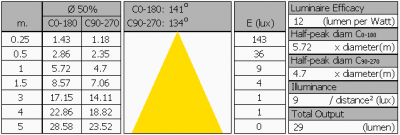The overview table is explained on the OliNo website.

Note: the minimum distance for which the E [lux] values are valid, is dependent on the layout of the strip. Laid out straightly over its 1000 mm then the minimum distance should be 5 x 1000 mm to be able to consider this light source as a point source and to be able to compute the Ev values at even bigger distances according to an inverse square law. However this is not pratical.

One other way to look at it is to observe 1 m of string length, which emits 29 lumen.

Now if it is needed to get a rough idea of the illuminance on a small distance, say 50 cm, then consider 1/5th of this distance of the lamp, here 10 cm. This part of the string will emit 1/50 * 36 lx = 7.2 lx (at 50 cm). Now the string is longer than that so the remainder will add luminance to it, but always less as it is further away. With help of a program like Dialux this can all be calculated, use the eulumdat file for that as given in the table above.

### EU Energy label classfication

With the measurement results of the luminous flux and the consumed power the classification on energy of this lamp is calculated. This information is requested in the EU for certain household lamps, see also the OliNo site that explains for which lamps it is requested, how the label looks like and what information it needs to contain.

Herewith the labels for this lamp in color and black and white.EU energy label of this lamp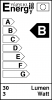Label in black and white.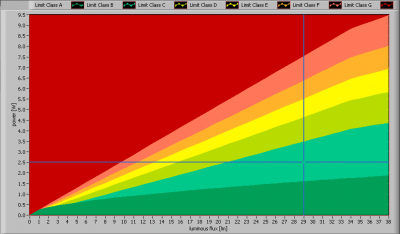The lamp’s performance in the lumen-Watt field, with the energy efficacy fields indicated.

### Eulumdat light diagram

This light diagram below comes from the program Qlumedit, that extracts these diagrams from an Eulumdat file. It is explained on the OliNo site.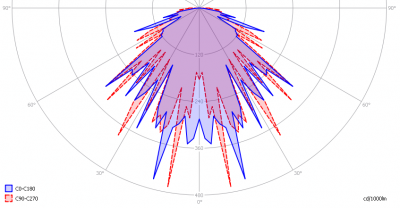The light diagram giving the radiation pattern.

It indicates the luminous intensity around the light bulb. The C0-C180 plane is along the width direction of the strip, meaning perpendicular to its length) and is the same as that of the C90-C270 plane (along the length direction of the strip).

The image shows many peaks, coming from the variation of the colors and intensity during the measurement.

### Illuminance Ev at 1 m distance, or luminous intensity Iv

Herewith the plot of the averaged luminous intensity Iv as a function of the inclination angle with the light bulb.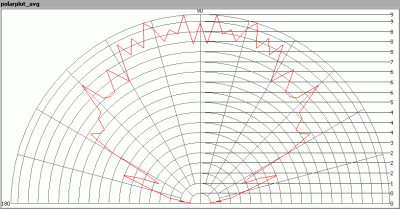The radiation pattern of the light bulb.

This radiation pattern is the average of the light output of the light diagram given earlier. Also, in this graph the luminous intensity is given in Cd.

These averaged values are used (later) to compute the lumen output.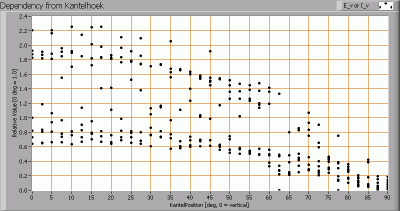Intensity data of every measured turn angle at each inclination angle.

This plot shows per inclination angle the intensity measurement results for each turn angle at that inclination angle. There normally are differences in illuminance values for different turn angles. However for further calculations the averaged values will be used.

When using the average values per inclination angle, the beam angle can be computed, being 141º for the C0-C180 and 133º for the C90-C270 plane.

### Luminous flux

With the averaged illuminance data at 1 m distance, taken from the graph showing the averaged radiation pattern, it is possible to compute the luminous flux.

The result of this computation for this light spot is a luminous flux of 29 Lm.

### Luminous efficacy

The luminous flux being 29 Lm, and the power of the light bulb being 2.5 W (this is the average value, the extremes were 1.9 W and 3.2 W), yields a luminous efficacy of 12 Lm/W.

### Electrical properties

A power factor of 0.44 means that for every 1 kWh net power consumed, a reactive component of 2.1 kVAr was needed.

 Lamp voltage 230 VAC Lamp current 25 mA Power P 2.5W Apparent power S 5.8 VA Power factor 0.44

Of this light bulb the voltage across ad the resulting current through it are measured and graphed. See the OliNo site how this is obtained.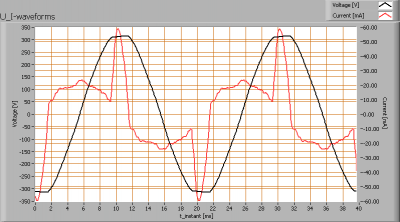Voltage across and current through the lightbulb

This waveforms have been checked on requirements posed by the norm IEC 61000-3-2:2006 (including up to A2:2009). See also the explanation on the OliNo website.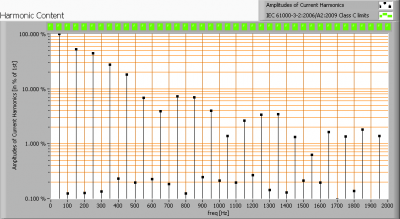Harmonics in in the current waveform and checked against IEC61000-3-2:2006 and A2:2009

There are no limits for the harmonics for lighting equipment <= 25 W.

The Total Harmonic Distortion of the current is computed as 78 %.

### Temperature measurements lamp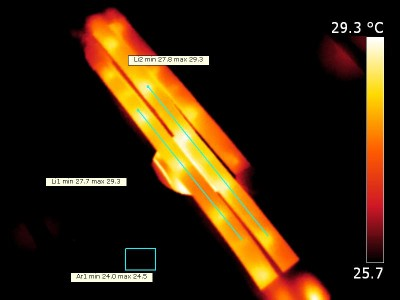Zoomed in on the ledstring

The emissivity of the silicone material is taken to be 0.95.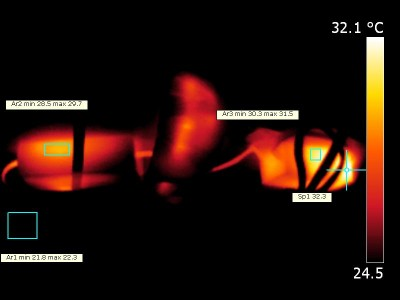The controller left and the power supply unit right

All these components have a rough surface so their emissivity is taken high, 0.95.

 status lamp > 2 hours on ambient temperature 24 deg C reflected background temperature 24 deg C camera Flir T335 emissivity 0.95(1) measurement distance 0.4 IFOVgeometric 0.136 mm per 0.1 m distance NETD (thermal sensitivity) 50 mK

(1) See the text for explanation.

### Color temperature and Spectral power distribution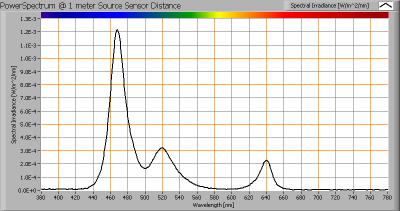The spectral power distribution of this light bulb, energies on y-axis valid at 1 m distance.

The measured color temperature is not measurable since most of the times the ledstring emits a colored light for which a correlated color temperature is not defined. The given spectrum here is the spectrum of a point in time, at a certain color.

### PAR value and PAR spectrum

To make a statement how well the light of this light bulb is for growing plants, the PAR-area needs to be determined. See the OliNo website how this all is determined and the explanation of the graph.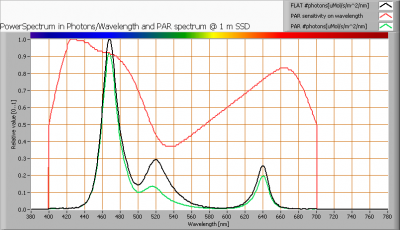The photon spectrum, then the sensitivity curve and as result the final PAR spectrum of the light of this light bulb

parameter value unit
PAR-number 0.15 μMol/s/m²
PAR-photon current 0.5 μMol/s
PAR-photon efficacy 0.2 μMol/s/W

The PAR efficiency is 77 % (valid for the PAR wave length range of 400 – 700 nm). So maximally 77 % of the total of photons in the light is effectively used by the average plant (since the plant might not take 100 % of the photons at the frequency where its relative sensitivity is 100 %).

### S/P ratio

The S/P ratio and measurement is explained on the OliNo website. Here the results are given.The power spectrum, sensitivity curves and resulting scotopic and photopic spectra (spectra energy content defined at 1 m distance).

The S/P ratio is 6.0. This is the value for this color (but the colors change).

More info on S/P ratio can be found on the OliNo website.

### Chromaticity diagram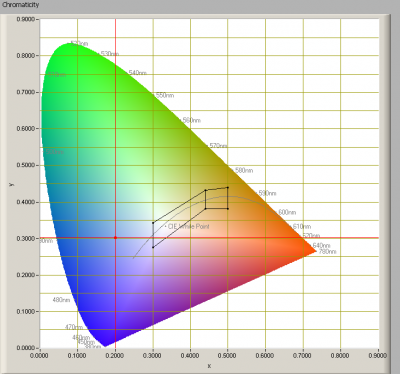The chromaticity space and the position of the lamp’s color coordinates in it.

The light coming from this lamp is outside the area of class A. This is an area defined for signal lamps, see also the OliNo website.

Its coordinates averaged are x=0.2003 and y=0.3023.

Herewith a number of measurements that show that the color point (chromaticity coordinates) is covering a wide area.

### Color Rendering Index (CRI) or also Ra

The CRI is not possible to calculate, as very often the chromaticity coordinates of the light is sofar from the Plackian locus that a CCT nor CRI can be calculated..

### Voltage dependency

The dependency of a number of lamp parameters on the lamp voltage is determined. For this, the lamp voltage has been varied and its effect on the following light bulb parameters measured: illuminance E_v [lx], the lamppower P [W] and the luminous efficacy [Lm/W].Lamp voltage dependencies of certain light bulb parameters, where the value at 230 V is taken as 100 %.

The illuminance and consumed power vary when the voltage is varied. This is mostly due to the fact that with changing colors the consumed power as well as the illuminance vary.

### Warm up effects

After switch on of a cold lamp, the effect of heating up of the lamp is measured on illuminance E_v [lx], the lamppower P [W] and the luminous efficacy [lm/W].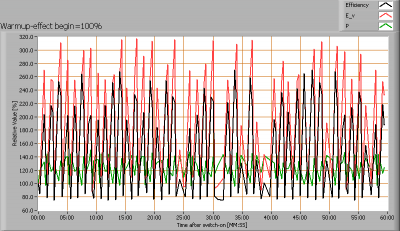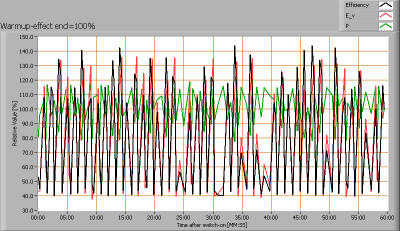Effect of warming up on different light bulb parameters. At top the 100 % level is put at begin, and at bottom at the end.

There are a lot of variations, due to the fact that the color is changing and therewith the amount of leds that are one and therewith the consumed power and illuminance. Over an hour, the variaitons do not have a tendency so assumed is that there is no warm up effect. This is likely since the power of 1.9 – 3.2 W is distributed over 30 leds and there is hardly any heat generated.

### Measure of flickering

An analysis is done on the measure of flickering of the light output by this light bulb. See the OliNo site for more information.

The measure of fast illuminance variation of the light of the light bulb

Depending on the color a number of illuminance modulation indexes were calculated.

parameter value unit
Flicker frequency 180 Hz
Illuminance modulation index 83, 8, 44, 22 %

The illuminance modulation index is computed as: (max_Ev – min_Ev) / (max_Ev + min_Ev).

The 180 Hz might be so high that modulation effects are not visible.

### Extra foto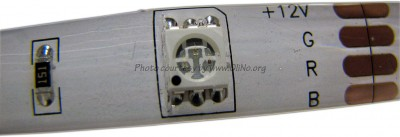Detail photo of the RGB led on the flexible printed circuit board